Courses

# RD Sharma Solutions -Ex-1.1 Number System, Class 9, Maths Class 9 Notes | EduRev

## Class 9 : RD Sharma Solutions -Ex-1.1 Number System, Class 9, Maths Class 9 Notes | EduRev

The document RD Sharma Solutions -Ex-1.1 Number System, Class 9, Maths Class 9 Notes | EduRev is a part of the Class 9 Course RD Sharma Solutions for Class 9 Mathematics.
All you need of Class 9 at this link: Class 9

Q1. Is 0 a rational number? Can you write it in the form P/Q , where P and Q are integers and Q ≠ 0?

Solution:

Yes, 0 is a rational number and it can be written in P÷Q form provided that Q ≠ 0

0 is an integer and it can be written various forms, for example

0÷2,0÷100,0÷95 etc.

Q2. Find five rational numbers between 1 and 2

Solution:

Given that to find out 5 rational numbers between 1 and 2

• Rational number lying between 1 and 2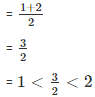• Rational number lying between 1 and 3/2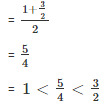• Rational number lying between 1 and 5/4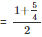Rational number lying between 3/2 and 2
= 9/2
= 1 < 9/8 < 5/4
• Rational number lying between 3/2 and 2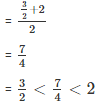• Rational number lying between 7/4 and 2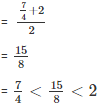Therefore,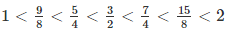Q3. Find out 6 rational numbers between 3 and 4

Solution:

Given that to find out 6 rational numbers between 3 and 4

We have,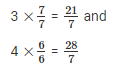We know 21 < 22 <  23 < 24 < 25 < 26 < 27 < 28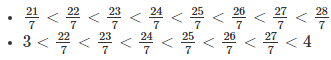Therefore, 6 rational numbers between 3 and 4 are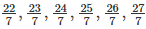Similarly to find 5 rational numbers between 3 and 4, multiply 3 and 4 respectively with 6/6 and in order to find 8 rational numbers between 3 and 4 multiply 3 and 4 respectively with 8/8 and so on.

Q4. Find 5 rational numbers between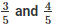Solution : Given to find out the 5 rational numbers between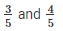To find 5 rational numbers between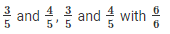We have,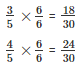We know  18 < 19 < 20 < 21 < 22 <  23 < 24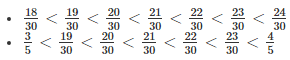Therefore, 5 rational numbers between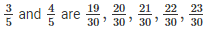Q5. Answer whether the following statements are true or false? Give reasons in support of your answer.

(i) Every whole number is a rational number

(ii) Every integer is a rational number

(iii) Every rational number is an integer

(iv) Every natural number is a whole number

(v) Every integer is a whole number

(vi) Every rational number is a whole number

Solution:

(i)  True. As whole numbers include and they can be represented

For example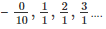. And so on.

(ii) True. As we know 1, 2, 3, 4 and so on, are integers and they can be represented in the form of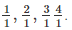(iii) False. Numbers such as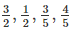are rational numbers but they are not integers.

(iv) True. Whole numbers include all of the natural numbers.

(v) False. As we know whole numbers are a part of integers.

(vi) False. Integers include -1, -2, -3 and so on….. .which is not whole number

Offer running on EduRev: Apply code STAYHOME200 to get INR 200 off on our premium plan EduRev Infinity!

91 docs

,

,

,

,

,

,

,

,

,

,

,

,

,

,

,

,

,

,

,

,

,

,

,

,

,

,

,

;